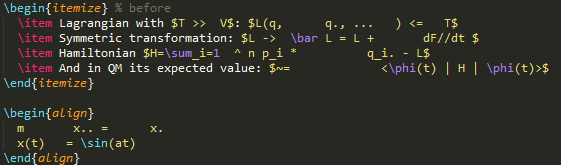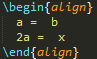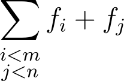Showing:

0

79

7yrs ago

1

0

UNKNOWN

# preTeX

preTeX is a Python (2 and 3) LaTeX preprocessor designed to make LaTeX syntax more concise and thereby the writing process faster and the code more readable. It consists of a number of Transformations, which are largely RegEx-powered replacements. It's focused on math. Examples in text and gif:

 in: The limit $\sum_ i=0 ^ N+1 q_i.. p. \frac a+b x^2-1$
out: The limit $\sum_{i=0}^{N+1} \ddot{q_i} \dot{p} \frac{a+b}{x^2-1}$## Why / Motivation

The math syntax of LaTeX is powerful and a defacto standard even outside of LaTeX. But it's ~30 years old and has some curiosities that make it awkward to transfer certain expressions from your brain into TeX code. For example it's almost completely whitespace agnostic. So \frac a+b 2 really is the same as \frac{a}{+}b2 and not the probably expected \frac{a+b}{2}. There's a macro system which can alleviate some of it, but it's still limited to the slash-and-{}-heavy tex syntax.

Starting with 1.0, preTeX is no longer safe as in: If you run it on random tex code, it sometimes changes things that can in theory be unwanted. This step makes it no longer a strict safe subset of LaTex. The reason for this was that this will realistically affect no one. Only extremely dense and dubious TeX code like a_bc d which is supposed to mean a_b c d and not a_{bc} d would be falsely interpreted. The upside of this change is that it greatly simplifies the internals and allows for even more expressive syntax.

## Usage

Install with (sudo) pip install pretex. The only mandatory argument is an input filename or string. You can also supply an output filename (default is {original}_t.tex) and change settings. Usage:

python pretex.py thesis.tex
python pretex.py thesis.tex -o thesis_output.tex
python pretex.py thesis.tex --set braket=disabled --set sub_superscript=aggressive
python pretex.py "a ... b"      #prints a \dots  b


It's fully tested with Python 2.7 to 3.4. Works in any math mode I know of. That is: $x$, $$x$$, $$x$$, $x$ for inline modes and in all of these math environments (starred and unstarred): equation, align, math, displaymath, eqnarray, gather, flalign, multiline, alignat.

Hint: This works well together with Pandoc, which makes it possible to mix LaTeX with Markdown code.

## HTML output

This is experimental and mostly used for debbuging right now. Enable with pretex --html .... Should write a filename_viz.html file in the sources directory that contains some highlighting and hover information.

## Transformations

nameinputoutputdefaultnotes
arrowa -> ba \to bon ✅below
approxa~=ba\approx bon ✅
leqa<=ba\leq bon ✅
geqa>=ba\geq bon ✅
lla<<ba\ll bon ✅
gga>>ba\gg bon ✅
neqa != ba \neq bon ✅
cdota*ba\cdot bon ✅below
dots1, 2, ...1, 2, \dotson ✅
braket<abc>\braket{a
frac\frac a+b 2\frac{a+b}{2}on ✅below
auto_alignon ✅below
substack \sum_{i<m \\ j<n}\sum_{\substack{i<m \\ j<n}}on ✅below
dotx..\ddot{x}off ❌below
sub_superscripte^a+be^{a+b}on ✅below
brackets(\frac 1 2)\left(\frac 1 2\right)off ❌below

### arrow

Simple arrow expressions like a -> b get replaced by their LaTeX counterpart a \to b. Note the necessary whitespace around it.

There is an extension to this when it comes to writing text over arrows. The LaTeX way to do this is 5 \xrightarrow{+3} 8. preTeX allows this to be written as 5 ->^{+3} 8. Note that this command requires the amsmath package to be included.

Both transformations are enabled by default. To only allow the first one, use pretex --set arrow=simple.

### auto_align

In an align(*) math environment when there is

1. 0 or 1 "=" on every line and
2. None of them is aligned by "&=" and
3. Two or more non-whitespace lines

Then they all get auto-aligned by replacing the = with &=. Also if there is no line separation with \\, it's added automatically for similar conditions. Only works on "sane" aligns, where there's no math on the same line as the begin and closing statements.### cdot

Works anywhere in math except for the case of a^* to prevent wrongful use in complex conjugation.

### braket

(This is about the Bra-ket notation from physics. Not to be confused with regular brackets)

A "natural" syntax for writing bras, kets, brakets and ketbras is supported. For |ket> and <bra| and |ket><bra|, there can't be any whitespace or curly braces in them and there have to be reasonable limits (space, braces, string start/end) before and after. That's because there is one tricky case where this could blow up: { x | x>0 }

There's also <a|b> or <a|b|c> for which the rules are a bit more relaxed (whitespace allowed inside). They all get translated into their respective \ket{}, \bra{} and \braket{} commands. Those are not included in vanilla LaTeX, but you could either use the LaTeX package braket which defines these, or define your own versions. Examples:

<a|b|c>      →  \braket{a|b|c}
|ket> <bra|  →  \ket{ket} \bra{bra}
|ke t>       →  |ke t>               % no whitespace inside!


### dot

Instead of writing \dot{a} for time derivations, just write a.. Same for \ddot and a... Works for some more complex structures, too. Examples:

x.          →  \dot{x}
f(q_i..)    →  f(\ddot{q_i})
\phi.       →  \dot{\phi}
\vec x.     →  \dot{\vec x}
\vec{abc}.  →  \dot{\vec{abc}}


Rule of thumb: The dot expression works with surrounding spaces or at the beginning/end inside braces.

Status: There is one use case that breaks this: Using punctuation in math mode. If you end a perfectly valid math expression with a dot and actually want to make a dot, this can make an unwanted change. Example: $a_i.$. That's why it's disabled by default at the moment. This was just one case out of ~5000 lines of tex code though, working on it. Enable with --set dot=enabled.

### sub_superscript

For relaxing the LaTeX rules with sub- or superscripting things with _ or ^. In default mode, what's being raised/lowered has to be alphanumeric, + or -. In particular it's unsafe to use backslashes, equal signs or brackets. That's to make sure that super tight notation like x^2+a_0 or ambiguous like \tau_\alpha stay untouched.

u_tt   →  u_{tt}
e^a+b  →  e^{a+b}
a_abc  →  a_{abc}


There is a more aggressive setting that allows even more relaxed expressions like

\tau_i=0        →  \tau{i=0}
a_i=0,j=0       →  a_{i=0,j=0}
a_\alpha,\beta  →  a_{\alpha,\beta}


That "aggressive" mode has to be enabled as a command line option (--set sub_superscript aggressive) and requires a space after the expression as a delimiter, even at the end of math mode! But allows anything inside except whitespace and curly brackets.

### frac

Instead of writing \frac{}{}, use spaces as delimiters.

\frac a+b c*d  →  \frac{a+b}{c*d}
\frac a+b 2    →  \frac{a+b}{2}


### substack

When typesetting a sum with two subscripted rows like:LaTeX doesn't allow this with normal newlines and you need to invoke the \substack command from amsmath this way: \sum_{\substack{i<m \\ j<n}}. preTeX does this for you, so you can just write \sum_{i<m \\ j<n}. Enabled by default, needs amsmath package.

### brackets

Automatically changes ()'s to their \left( and \right) versions when they're not already like that. This can be typographically unwanted, so it's disabled by default. Activate with pretex -set brackets=enabled ...

• braket-size would be neat to be able to set. Right now they default to the small versions (\ket etc). There are big versions (\Ket) but I have no clue what's a clever way to indicate their use in the code. Right now that's a config var, but that's global or too much effort for a per-use-case
• Verbose mode that reports changes
• auto-insert \linebreak` in inline math after punctuation and forced whitespace?

## Rate & Review

Great Documentation0
Easy to Use0
Performant0
Highly Customizable0
Bleeding Edge0
Responsive Maintainers0
Poor Documentation0
Hard to Use0
Slow0
Buggy0
Abandoned0
Unwelcoming Community0
100No reviews found
Be the first to rate

## AlternativesNo alternatives found

## TutorialsNo tutorials found